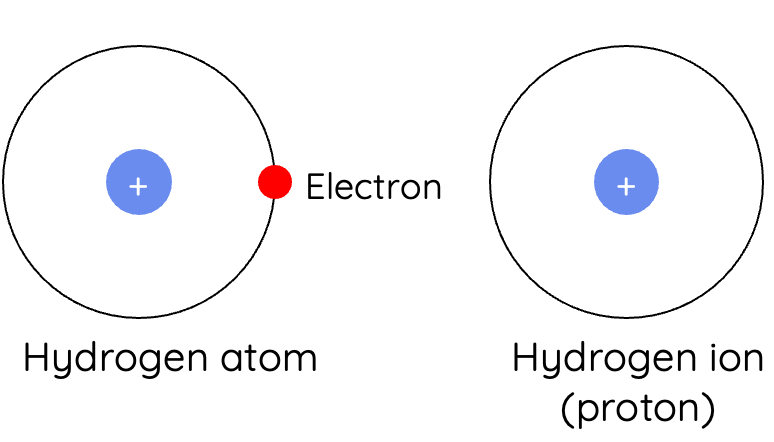# Acid-Base Reactions

This is part of Year 11 HSC Chemistry course under the topic of Chemical Reactions

### HSC Chemistry Syllabus

• Conduct investigations to predict and identify the products of a range of reactions, for example:
• Acid/Base Reactions
• Acid/Carbonate Reactions

### Acid-Base Reactions

This video discusses fundamentals of acid-base reactions including basic acid-base reactions such as metal/acid and acid/carbonate reactions.

### What are Acids and Bases?

In the context of the NSW HSC Chemistry syllabus, acids and bases are defined by two prominent theories; The Arrhenius, and Brønsted - Lowry theories of acids and bases.

Arrhenius Acid

• According to the Arrhenius acid-base theory, an Arrhenius acid is a compound that dissociates in water to produce the hydrogen ion H^+. For instance, hydrochloric acid dissociates into hydrogen and chloride Cl^– ions and hence it is classified as an Arrhenius acid.

Arrhenius Base

• An Arrhenius Base, similar to an Arrhenius acid, is a compound which dissociates to yield the hydroxide ion OH^–. An example of on such compound is sodium hydroxide which dissociates to form sodium Na^+ and hydroxide ions.

Brønsted-Lowry Acid-Base Theory:

• The Brønsted-Lowry acid-base theory defines an acid as a proton donor i.e., a hydrogen ion donor, while a base acts as a proton acceptor.The figure above demonstrates how hydrogen ions are simply protons. Hydrogen atoms consist of a proton and an electron, which when losing an electron to form an ion, leaves just a proton behind.

Arrhenius and Brønsted-Lowry bases are explored in further detail in Year 12 Module 6 "Acids and Bases" of the NSW HSC Chemistry Syllabus.

### Neutralisation

A neutralisation reaction is a type of chemical reaction in which an acid and a base react quantitatively together to produce a salt and water.

$$\text{Acid} + \text{base} \rightarrow \text{water}$$

Under the model of the Arrhenius acid-base theory, the process of neutralisation takes place when an acid and a base react together, resulting in the formation of water due to the interaction between H^+ and OH^– ions. The net ionic equation for Arrhenius neutralisation is:

$$H^+(aq) + OH^–(aq) \rightarrow H_2O(l)$$

Under the model of the Brønsted-Lowry acid-base theory, neutralisation takes place when an acid donates a hydrogen ion to a base which accepts it. To illustrate this, consider the reaction between hydrochloric acid and ammonia.

$$HCl(aq) + NH_3(aq) \rightarrow NH_4^+(aq) + Cl^–(aq)$$

In this reaction, hydrochloric acid ionises in to form the hydrogen and chloride ions. When the solution of ammonia is added it accepts a hydrogen ion which was donated by the hydrochloric acid and forms the ammonium ion NH_4^+.

### Acid-Metal Reactions:

These reactions can be generalised as:

$$\text{Acid} + \text{Metal} \rightarrow \text{Salt} + \text{Hydrogen Gas}$$

Acid-metal reactions are still considered as acid-base reactions because of the formation of hydroxide when metals react with water.

The stepwise reactions between sodium metal and aqueous hydrochloric acid are demonstrated by the following equations.

Step 1: Sodium reacts with water to form sodium hydroxide and hydrogen gas

$$2Na(s) + 2H_2O(l) \rightarrow 2NaOH(aq) + H_2(g)$$

Step 2: Sodium hydroxide then reacts in a neutralisation reaction with hydrochloric acid

$$NaOH(aq) + HCl(aq) \rightarrow NaCl(aq) + H_2O(l)$$

Overall Equation:

$$2Na(s) + 2HCl(aq) \rightarrow H_2(g) + 2NaCl(aq)$$

Note: Consider sodium's reaction with water which forms sodium hydroxide. This then reacts with hydrochloric acid resulting in salt and water. The aqueous nature indicates that water is not included in the reaction.

Quick test: The pop test can be used to verify acid and metal reactions. Combusting small amounts of hydrogen gas results in a squeaky sound, signalling the production of water – a highly exothermic process.

### Reactions of Acids with Metal Oxides and Carbonates:

1. Acids and Metal Oxides

Reactions between acids and metal oxides generally result in the formation of salt and water. Initially the reaction forms a hydroxide when the oxide reacts with water. This hydroxide then reacts with the acid to produce salt and water.

E.g. reaction between calcium oxide and hydrochloric acid

$$CaO(s) + 2HCl(aq) \rightarrow CaCl_2(aq) + H_2O(l)$$

Steps:

$$CaO(s) + H_2O(l) \rightarrow Ca(OH)_2(aq)$$

$$Ca(OH)_2(aq) + 2HCl(aq) \rightarrow CaCl_2(aq) + 2H_2O(l)$$

2. Acids and Carbonates:

These are a vital type of reaction which is worth memorising. An acid reacting with a carbonate gives rise to a salt, water, and carbon dioxide. Consider the reaction between hydrochloric acid and calcium carbonate.

$$2HCl(aq) + CaCO_3(aq) \rightarrow CaCl_2(aq) + H_2O(l) + CO_2(g)$$